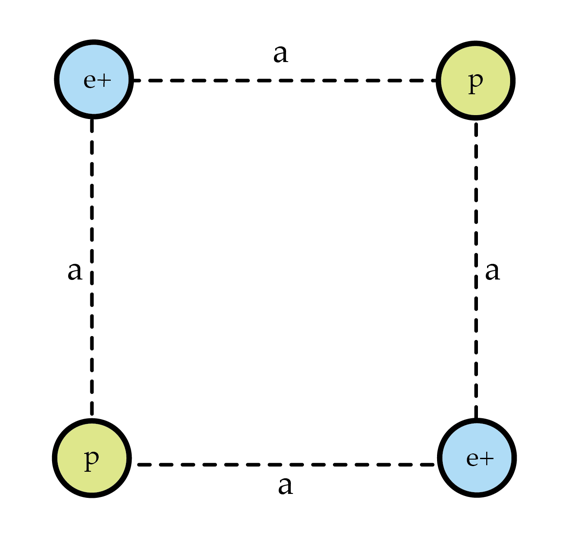# Scattering protons and positrons

Two protons and two positrons form a square as shown in the figure. All the particles are initially at rest. Due to Coulomb's repulsion, the particles fly off to infinity. Determine the ratio $\frac{v_{p}}{v_{e}}$ where $v_{p}$ and $v_{e}$ are the final velocities of the protons and positrons, respectively. Note that the positron, being electron's antiparticle, has the same mass as the electron and opposite charge $(q=+1.6\times 10^{-19}~\textrm{C})$.Details and assumptions

• $\frac{m_{p}}{m_{e}}=1836$
• Hint: since the protons are so much heavier, you can do this without having to solve momentum conservation. Think about which particles fly away first...
×